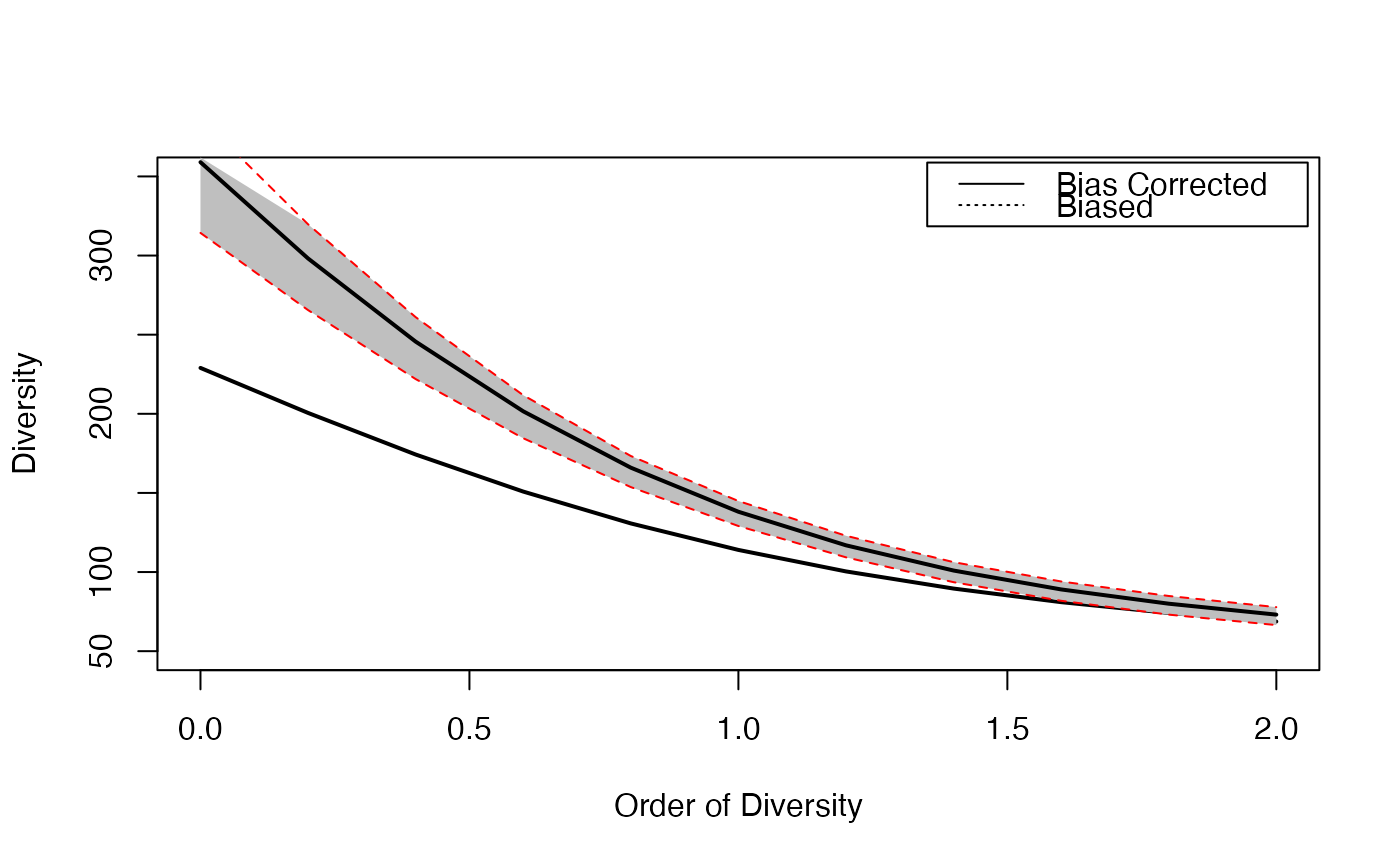Calculates the diversity or entropy profile of a community, applying a community function to a vector of orders.

CommunityProfile(FUN, NorP, q.seq = seq(0, 2, 0.1),
NumberOfSimulations = 0, Alpha = 0.05, BootstrapMethod = "Chao2015",
size = 1, ..., ShowProgressBar = TRUE, CheckArguments = TRUE)
as.CommunityProfile(x, y, low = NULL, high = NULL, mid = NULL)
is.CommunityProfile(x)
# S3 method for CommunityProfile
plot(x, ..., main = NULL,
xlab = "Order of Diversity", ylab = "Diversity", ylim = NULL,
LineWidth = 2, ShadeColor = "grey75", BorderColor = "red")
# S3 method for CommunityProfile
autoplot(object, ..., main = NULL,
xlab = "Order of Diversity", ylab = "Diversity",
ShadeColor = "grey75", alpha = 0.3, BorderColor = "red",
col = ggplot2::GeomLine$default_aes$colour,
lty = ggplot2::GeomLine$default_aes$linetype,
lwd = ggplot2::GeomLine$default_aes$size)
CEnvelope(Profile, LineWidth = 2, ShadeColor = "grey75", BorderColor = "red", ...)

## Arguments

FUN

The function to be applied to each value of $$q.seq$$. Any function accepting a numeric vector (or a two-column matrix) and a number as first two arguments and an argument named CheckArguments is acceptable (other arguments of the functions are passed by ...). See *Details* for useful entropy and diversity functions and *Examples* for an ad-hoc one.

NorP

A numeric vector. Contains either abundances or probabilities.

q.seq

A numeric vector: the sequence of diversity orders to address. Default is from 0 to 2.

NumberOfSimulations

The number of simulations to run, 0 by default.

Alpha

The risk level, 5% by default.

BootstrapMethod

The method used to obtain the probabilities to generate bootstrapped communities from observed abundances. See rCommunity.

size

The size of simulated communities used to compute the bootstrap confidence envelope. 1 (default) means that the actual size must be used.

object

An object.

x

An object to be tested or plotted or the vector of orders of community profiles in as.CommunityProfile.

y

Entropy or diversity values of each order, corresponding to x values.

low

Entropy or diversity lower bound of the confidence envelope, corresponding to x values.

high

Entropy or diversity higher bound of the confidence envelope, corresponding to x values.

mid

Entropy or diversity center value (usually the mean) of the confidence envelope, corresponding to x values.

Profile

An CommunityProfile to be plotted.

...

Additional arguments to be passed to FUN in CommunityProfile, to plot in plot.CommunityProfile or to lines in CEnvelope.

main

The main title of the plot.

xlab

The x axis label of the plots.

ylab

The y axis label of the plot.

ylim

The interval of y values plotted.

LineWidth

The width of the line that represents the actual profile.

The color of the shaded confidence envelope.

BorderColor

The color of the bounds of the confidence envelope.

alpha

Opacity of the confidence enveloppe, between 0 and 1.

col

The color of the geom objects. See "Color Specification" in par.

lty

The type of the lines. See lines.

lwd

The width of the lines. See lines.

ShowProgressBar

If TRUE (default), a progress bar is shown.

CheckArguments

Logical; if TRUE, the function arguments are verified. Should be set to FALSE to save time when the arguments have been checked elsewhere.

## Details

The function CommunityProfile is used to calculate diversity or entropy profiles based on community functions such as Tsallis or ChaoPD. The first two arguments of the function must be a probability or abundance vector and a number ($$q$$). Additional arguments cannot be checked. Unexpected results may be returned if FUN is not used properly.

If NumberOfSimulations is greater than 0, a bootstrap confidence interval is produced by simulating communities with rCommunity and calculating their profiles. The size of those communities may be that of the actual community or specified by size. Simulating communities implies a downward bias in the estimation: rare species of the actual community may have abundance zero in simulated communities. Simulated diversity values are recentered if size = 1 so that their mean is that of the actual community. Else, it is assumed that the bias is of interest and must not be corrected.

CommunityProfile objects can be plotted. They can also be added to the current plot by CEnvelope.

## Value

A CommunityProfile, which is a list:

x

The order $$q$$ values

y

The entropy or diversity values returned by FUN

low

The lower bound of the confidence interval

high

The upper bound of the confidence interval

## Author

Eric Marcon <Eric.Marcon@agroparistech.fr>, Bruno Herault <Bruno.Herault@cirad.fr>

## Examples

# Load Paracou data (number of trees per species in two 1-ha plot of a tropical forest)
data(Paracou618)
# Plot diversity estimated without bias correction
plot(CommunityProfile(Diversity, Paracou618.MC$Ps, seq(0, 2, 0.2)), lty=3, ylim=c(50, 350)) # Estimate diversity, with a condidence envelope # (only 10 simulations to save time, should be 1000) Profile <- CommunityProfile(Diversity, as.AbdVector(Paracou618.MC$Ns),
seq(0, 2, 0.2), Correction="UnveilJ", NumberOfSimulations=10)
#>
# Complete the plot, and add the legend
CEnvelope(Profile, main="Paracou Plots Diversity")
legend("topright", c("Bias Corrected", "Biased"), lty=c(1,3), inset=0.01)# Advanced use with beta-diversity functions :
# Profile of the beta entropy of the first community of Paracou618.
# Observed and expected probabilities are bound into a 2-column matrix
# An intermediate function is necessary to separate them before calling TsallisBeta
# The CheckArguments is mandatory but does not need to be set: CommunityProfile() sets it to FALSE
CommunityProfile(function(PandPexp, q, CheckArguments)
{TsallisBeta(PandPexp[, 1], PandPexp[, 2], q)},
NorP=cbind(Paracou618.MC$Psi[, 1], Paracou618.MC$Ps), q.seq=seq(0, 2, 0.2))
#> $x #>  0.0 0.2 0.4 0.6 0.8 1.0 1.2 1.4 1.6 1.8 2.0 #> #>$y
#>   53.42961065 16.60496236  5.55701448  2.02871915  0.80974134  0.34993577
#>    0.16100624  0.07750741  0.03849038  0.01952232  0.01004705
#>
#> attr(,"class")
#>  "CommunityProfile"